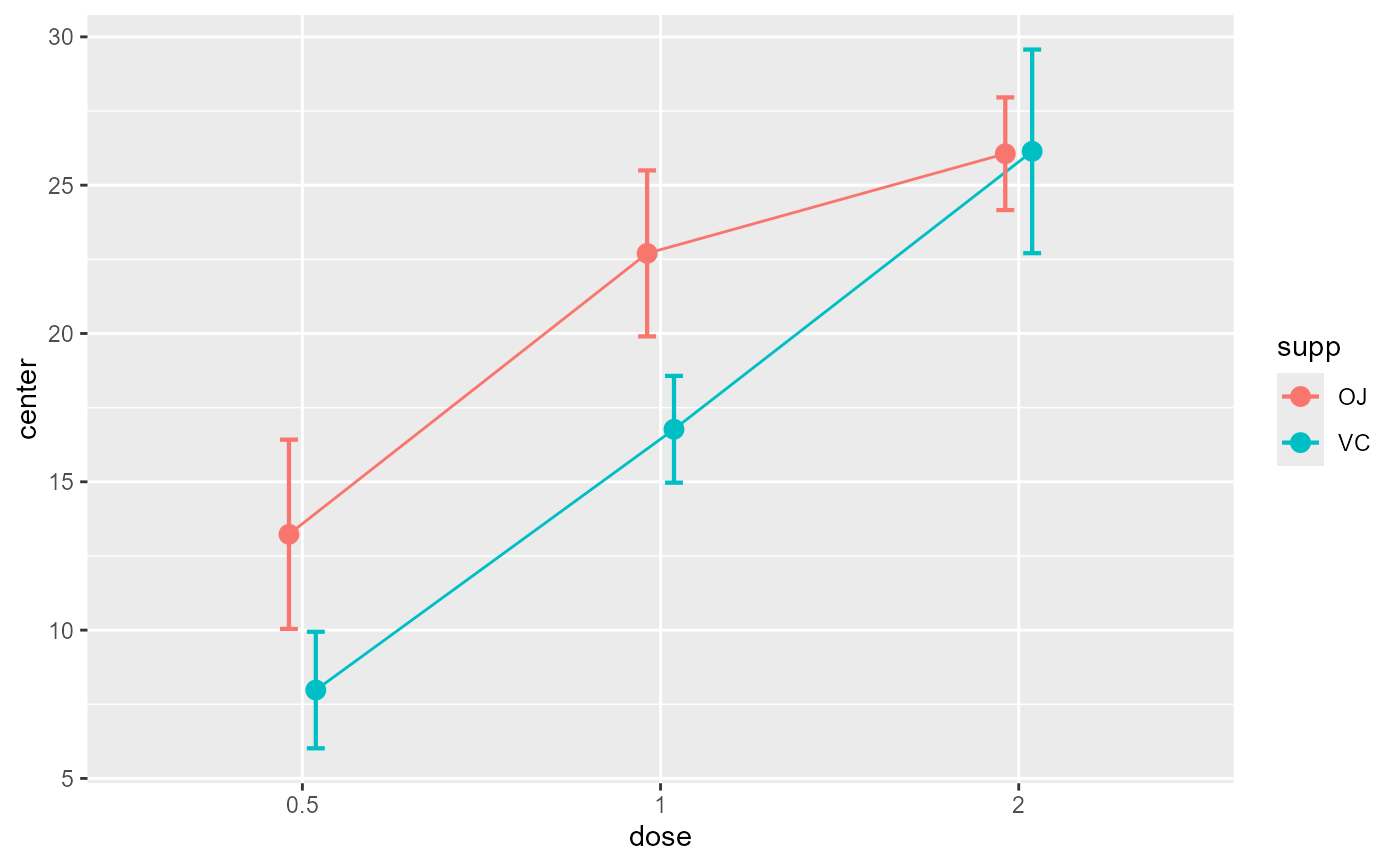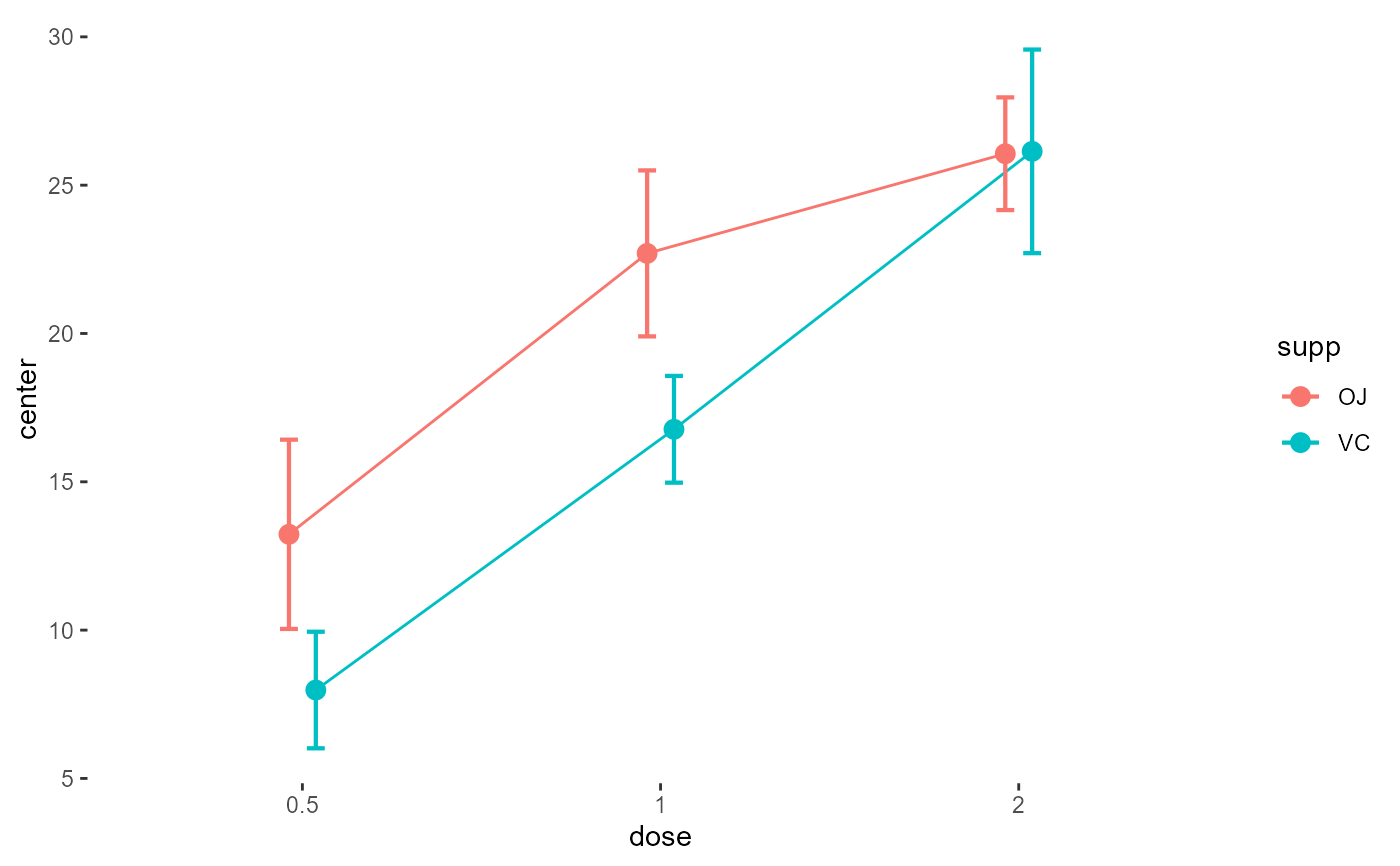makeTransparent is an extension to ggplots which makes all the elements of the plot transparent except the data being displayed. This is useful to superimpose multiple plots, e.g. to generate plots with multiple error bars for example.

makeTransparent()

## Value

does not return anything; set the elements to transparent.

## Examples


# make a basic plot
superbPlot(ToothGrowth, BSFactors = c("dose", "supp"),
variables = "len")# make a basic plot with transparent elements
superbPlot(ToothGrowth, BSFactors = c("dose", "supp"),
variables = "len") + makeTransparent()